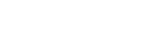Creating Quizzes for Descriptive Statistics - Maple Help

Home : Support : Online Help : Applications and Example Worksheets : Grading and Assessment : Creating Quizzes for Descriptive Statistics

Student Statistics: Creating Quizzes for Descriptive Statistics

Using the Quiz command from the Grading package, it is possible to generate interactive quizzes that ask students to answer questions on topics in Statistics, such as finding the mean, median or standard deviation of a sample of data, or specifying the interquartile range directly from the plot of a sample.Defining a randomly generated data sample

To begin with, declare a procedure that generates a random sample of data:

 > InitializeData := proc()     local data;          randomize();     data := Vector[row](5, rand(1 .. 10));     Grading:-Quiz:-Set(= data);     return data; end proc:

Two key commands are used to randomly generate the data: randomize, which resets the seed for random number generation and inside of the data vector, and the rand( ) constructor, which is a random number generator, generating numbers between 1 and 10 in this case.

In order to use this data sample in a quiz, use the Grading[Quiz][Set] command to send the generated data sample to the quiz creation module. Once this procedure is created, it can be called to return a randomly generated sample:

 > InitializeData();Quiz: Calculate the Mean

 > GradeMean := proc()     uses Grading:-Quiz;     evalb( Student:-Statistics:-Mean( Get() ) = Get($RESPONSE) ); end proc: In this procedure, the known value of the sample is compared using the Student[Statistics][Mean] command where the response is entered by the student. The response is retrieved using the Grading[Quiz][Get] command. Use the Quiz command to generate the quiz by first specifying the question, then grading procedure, and finally any initializing procedures used to generate the data:  > Grading:-Quiz( "Find the mean of this sample:$data", GradeMean, InitializeData );

 Find the mean of this sample:By entering a value in the above text box and clicking 'Check Answer', students will receive a notification on whether or not their response is correct.

It is also important to note that all of the created quizzes can be regenerated using the 'Try Another' button in the created quiz.Quiz: Calculate the Median

Similar to the example for finding the Mean, since a procedure for initializing the data has already been created, start by creating the grading procedure for comparing the known Median value with the entered response. The evalb command is used to determine if these two match, returning either a true or false answer.

 > GradeMedian := proc()     uses Grading:-Quiz;     evalb( Student:-Statistics:-Median( Get() ) = Get($RESPONSE) ); end proc:  > Grading:-Quiz( "Find the median of this sample:$data", GradeMedian, InitializeData );

 Find the median of this sample:By entering a value in the above text box and clicking 'Check Answer', students will receive a notification on whether or not their response is correct.

It is also important to note that all of the created quizzes can be regenerated using the 'Try Another' button in the created quiz.Quiz: Calculate the Standard Deviation

 > GradeStandardDeviation := proc()     uses Grading:-Quiz;     evalb( Student:-Statistics:-StandardDeviation( Get() ) = Get($RESPONSE) ); end proc:  > Grading:-Quiz( "Find the standard deviation of this sample:$data", GradeStandardDeviation, InitializeData );

 Find the standard deviation of this sample:By entering a value in the above text box and clicking 'Check Answer', students will receive a notification on whether or not their response is correct.

It is also important to note that all of the created quizzes can be regenerated using the 'Try Another' button in the created quiz.Quiz: Find the Interquartile Range

The Quiz command can also be used to create questions using plots. In the following example, a new initialization procedure is created that generates a random sample of data and in addition, a plot of this data. Both of these are set to variable names that are then sent to the quiz module using the Quiz[Set] command.

 > InitPlot := proc()     local data, qplot;          randomize();     data := Vector[row](5, rand(1 .. 10));     Grading:-Quiz:-Set(= data);     qplot := Student:-Statistics:-InterquartileRange(data, output = plot);     Grading:-Quiz:-Set(= qplot);     return NULL; end proc:

Similar to the examples above, the grading procedure for the plot question compares a response with the calculated value from routines in Student[Statistics]:

 > GradeIQR := proc()     uses Grading:-Quiz;     evalb( Student:-Statistics:-InterquartileRange( Get() ) = Get($RESPONSE) ); end proc: In this case, you can also add more options to the quiz command, such as specifying the size of the plot:  > Grading:-Quiz( "Using the following graph, calculate the interquartile range:$plot", GradeIQR, InitPlot, plotsize=[400,400] );

 Using the following graph, calculate the interquartile range:>

By entering a value in the above text box and clicking 'Check Answer', students will receive a notification on whether or not their response is correct.

It is also important to note that all of the created quizzes can be regenerated using the 'Try Another' button in the created quiz.See Also